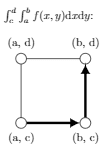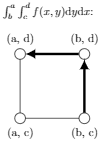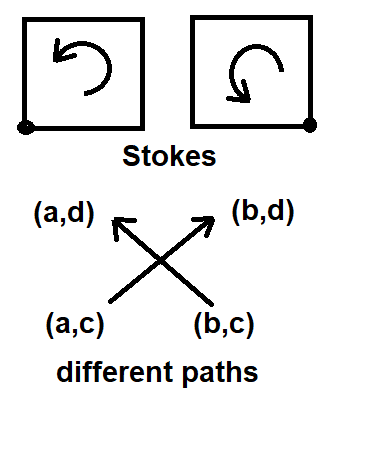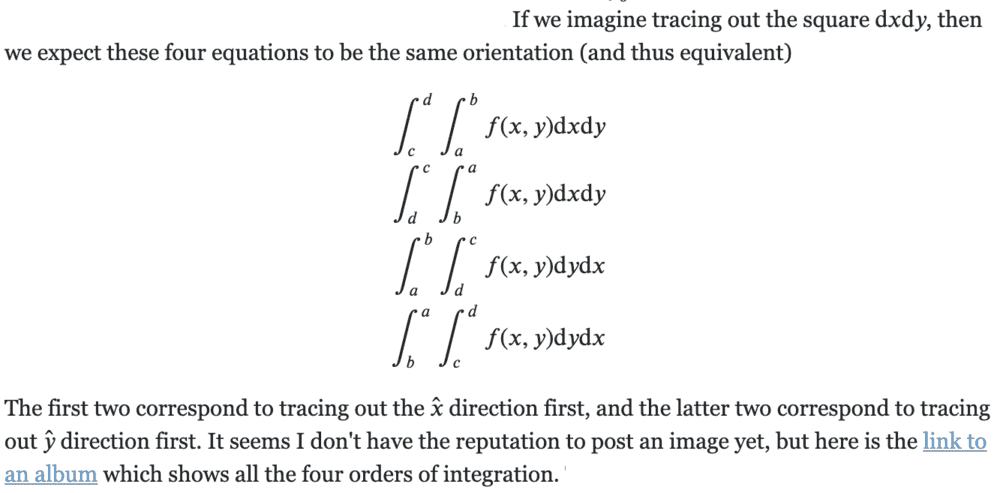# Orientation of double integrals

• B
I learned that, because ##du \, dv = \frac{\partial(u,v)}{\partial(x,y)} dx \, dy##, if you set ##u=y## and ##v=x## then you get that ##dx \, dy = - dy \, dx##. And that the product of two differentials is a wedge product, which is antisymmetric. If coordinates are orthogonal, then ##dx \, dy = dx \wedge dy = - dy \wedge dx = - dy \, dx##.

Now take a double integral, over the unit square:$$\int_0^1 \left( \int_0^1f(x,y) dy \right) dx = \int_C f(x,y) (dx \wedge dy) = \int_0^1 \left( \int_0^1f(x,y) dx \right) dy$$The two integrals on either side have the same orientation; what does this mean? I'm guessing it has to do with whether you go around the integration path clockwise or anti-clockwise in the ##x##-##y## space, but how do you judge this from the limits on the integral?

Last edited by a moderator:

Mentor
2022 Award
The orientation of integration is from bottom to top. And you already used an abbreviation:
$$\int_a^b \left(\int_c^d f(x,y)\,dy\right)\,dx = \int_{x=a}^{x=b} \left(\int_{y=c}^{y=d} f(x,y)\,dy\right)\,dx$$
This is sometimes important, because ##a,b;c,d## can change if you swap integration order, since the variables are not always independent. Orientation is already given in the basic case: ##\int_0^1 = -\int_1^0.## Orientation in physical three dimensional problems is usually denoted by whether the normal vector of a surface points in- or outward.

•etotheipi
Thanks. I saw one explanation on MSE (link here) which claimed that the following two integrals should be equal because they both "go anticlockwise".I'm not sure if that's correct.

Last edited by a moderator:
Mentor
2022 Award
If you integrate over the entire area with the help of Stokes theorem, then both are equally oriented. If you only integrate half of the paths, from ##(a,c)## to ##(b,d)## or from ##(b,c)## to ##(a,d)##, then you get different signs:Try a parameterization of the integration paths in your examples and integrate along one variable.

•etotheipi
If you integrate over the entire area with the help of Stokes theorem

Is there a version of Stokes' theorem that applies to scalar fields?

Mentor
2022 Award
I had to look it up. They are all just another version of the fundamental theorem of calculus, adjusted to more dimensions and manifolds. Physicists probably know them better.
Cauchy-Goursat is for complex functions, and Gauß' divergence theorem for vector fields. A scalar field is just a vector field where the values are one dimensional vectors.
cp. https://www.physicsforums.com/insights/pantheon-derivatives-part-v/

You can find example in the solution manuals of our challenge threads:

Thanks, I'll start reading the insight in a second!

Try a parameterization of the integration paths in your examples and integrate along one variable.

I'm getting a little bit stuck with this. For a surface integral, I thought we required 2 coordinates to parameterise the surface. I wondered what you mean by an 'integration path' in the context of a surface integral, and how we can reduce it to a 1 coordinate parameterisation?$$\int_c^d \int_a^b f(x,y) dx dy$$

Mentor
2022 Award
Yes, you need two parameters for a surface, or Stokes to reduce the problem to the boundary, which is why the surface integral equals ##\int_0^1\int_0^1f(x,y)\,dx\,dy##. The orientation is determined by ##[0,1]\times [0,1]## as opposed to ##[0,1]\times [1,0]##.

In one dimension we have ##\int_a^b f(x)\,dx = F(b)-F(a)## or if the path is closed ##0= \int_a^a f(x)\,dx =\int_a^b f(x)\,dx +\int_b^a f(x)\,dx.##

However, if you only integrate along the vectors without going back and thus double the minus signs, then it is a line integral ##\int_{(a,c)}^{(b,d)} f(\vec{x})\,d\vec{x}\neq \int_{(b,c)}^{(a,d)} f(\vec{x})\,d\vec{x}## with two different parameterizations.

•etotheipi
Mentor
2022 Award
The orientation of the surfaces (squares) in your example are given by ##(1,0,0) \times (0,1,0)## on the left and ##(0,1,0)\times (-1,0,0)## on the right, which are equally ##(0,0,1).##

Staff Emeritus
Gold Member
I feel like the picture was just trying to get at the reverse of one of the x and y directions of integration when you do dydx, and it confused fresh whether you were talking about a path integral around the boundary or something.

Mentor
2022 Award
I think the discussion is about the question: "Why does swapping integration order does not swap the sign while ##dx\wedge dy = -dy\wedge dx\,##?" And the answer is, that we did not swap the signs of combined length and height (see the calculation in post #9) in the first case (Fubini), but did so in the second (Graßmann).

•etotheipi
I think I understand it slightly better now. I was just trying to understand what this meant:because it seems to say all 4 are equivalent, but to me at least they are not.

Mentor
2022 Award
I think I understand it slightly better now. I was just trying to understand what this meant:

View attachment 269402

because it seems to say all 4 are equivalent, but to me at least they are not.
The first two are equivalent and the last two are, assuming that the function allows changing the order of integration.

Edit: I usually add such MS paint pictures as above (quick and dirty, and small) via the buffer: Ctrl+A,Ctrl+C,Ctrl+V.

•etotheipi
Yeah, that's what I had in mind. We start off with the multiple integral over the region ##R = [a,b] \times [c,d]##,$$I = \int_R f(x,y) \, d(x,y)$$Then Fubini allows us to re-write this as an iterated integral,$$I = \int_{c}^{d} \left( \int_{a}^{b} f(x,y) dx \right) dy = \int_{a}^{b} \left( \int_{c}^{d} f(x,y) dy \right) dx$$where I've put in the brackets, but they're often left out. Once in this form, we can just use the property that ##\int_a^b f(x) dx = -\int_b^a f(x) dx## to obtain other equivalent expressions, e.g.$$I = \int_d^c \left( \int_b^a f(x,y) dx \right) dy$$

Last edited by a moderator: